# E7 (mathematics)

In mathematics, E7 is the name of several closely related Lie groups, linear algebraic groups or their Lie algebras e7, all of which have dimension 133; the same notation E7 is used for the corresponding root lattice, which has rank 7. The designation E7 comes from the Cartan–Killing classification of the complex simple Lie algebras, which fall into four infinite series labeled An, Bn, Cn, Dn, and five exceptional cases labeled E6, E7, E8, F4, and G2. The E7 algebra is thus one of the five exceptional cases.

The fundamental group of the (adjoint) complex form, compact real form, or any algebraic version of E7 is the cyclic group Z/2Z, and its outer automorphism group is the trivial group. The dimension of its fundamental representation is 56.

## Real and complex forms

There is a unique complex Lie algebra of type E7, corresponding to a complex group of complex dimension 133. The complex adjoint Lie group E7 of complex dimension 133 can be considered as a simple real Lie group of real dimension 266. This has fundamental group Z/2Z, has maximal compact subgroup the compact form (see below) of E7, and has an outer automorphism group of order 2 generated by complex conjugation.

As well as the complex Lie group of type E7, there are four real forms of the Lie algebra, and correspondingly four real forms of the group with trivial center (all of which have an algebraic double cover, and three of which have further non-algebraic covers, giving further real forms), all of real dimension 133, as follows:

• The compact form (which is usually the one meant if no other information is given), which has fundamental group Z/2Z and has trivial outer automorphism group.
• The split form, EV (or E7(7)), which has maximal compact subgroup SU(8)/{±1}, fundamental group cyclic of order 4 and outer automorphism group of order 2.
• EVI (or E7(-5)), which has maximal compact subgroup SU(2)·SO(12)/(center), fundamental group non-cyclic of order 4 and trivial outer automorphism group.
• EVII (or E7(-25)), which has maximal compact subgroup SO(2)·E6/(center), infinite cyclic findamental group and outer automorphism group of order 2.

For a complete list of real forms of simple Lie algebras, see the list of simple Lie groups.

The compact real form of E7 is the isometry group of the 64-dimensional exceptional compact Riemannian symmetric space EVI (in Cartan's classification). It is known informally as the "quateroctonionic projective plane" because it can be built using an algebra that is the tensor product of the quaternions and the octonions, and is also known as a Rosenfeld projective plane, though it does not obey the usual axioms of a projective plane. This can be seen systematically using a construction known as the magic square, due to Hans Freudenthal and Jacques Tits.

The Tits–Koecher construction produces forms of the E7 Lie algebra from Albert algebras, 27-dimensional exceptional Jordan algebras.

## E7 as an algebraic group

By means of a Chevalley basis for the Lie algebra, one can define E7 as a linear algebraic group over the integers and, consequently, over any commutative ring and in particular over any field: this defines the so-called split (sometimes also known as “untwisted”) adjoint form of E7. Over an algebraically closed field, this and its double cover are the only forms; however, over other fields, there are often many other forms, or “twists” of E7, which are classified in the general framework of Galois cohomology (over a perfect field k) by the set H1(k, Aut(E7)) which, because the Dynkin diagram of E7 (see below) has no automorphisms, coincides with H1(k, E7, ad).

Over the field of real numbers, the real component of the identity of these algebraically twisted forms of E7 coincide with the three real Lie groups mentioned above, but with a subtlety concerning the fundamental group: all adjoint forms of E7 have fundamental group Z/2Z in the sense of algebraic geometry, meaning that they admit exactly one double cover; the further non-compact real Lie group forms of E7 are therefore not algebraic and admit no faithful finite-dimensional representations.

Over finite fields, the Lang–Steinberg theorem implies that H1(k, E7) = 0, meaning that E7 has no twisted forms: see below.

## Algebra

### Dynkin diagram

The Dynkin diagram for E7 is given by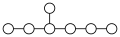.

### Root system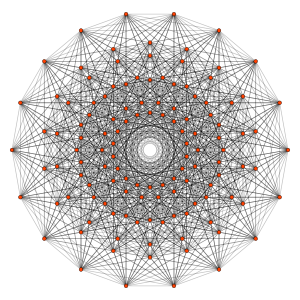The 126 vertices of the 231 polytope represent the root vectors of E7, as shown in this Coxeter plane projection
Coxeter–Dynkin diagram: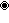Even though the roots span a 7-dimensional space, it is more symmetric and convenient to represent them as vectors lying in a 7-dimensional subspace of an 8-dimensional vector space.

The roots are all the 8×7 permutations of (1,−1,0,0,0,0,0,0) and all thepermutations of (½,½,½,½,−½,−½,−½,−½)

Note that the 7-dimensional subspace is the subspace where the sum of all the eight coordinates is zero. There are 126 roots.

The simple roots are

(0,−1,1,0,0,0,0,0)
(0,0,−1,1,0,0,0,0)
(0,0,0,−1,1,0,0,0)
(0,0,0,0,−1,1,0,0)
(0,0,0,0,0,−1,1,0)
(0,0,0,0,0,0,−1,1)
(½,½,½,½,−½,−½,−½,−½)

We have ordered them so that their corresponding nodes in the Dynkin diagram are ordered from left to right (in the diagram depicted above) with the side node last.

#### An alternative description

An alternative (7-dimensional) description of the root system, which is useful in considering E7 × SU(2) as a subgroup of E8, is the following:

Allpermutations of (±1,±1,0,0,0,0,0) preserving the zero at the last entry, all of the following roots with an even number of +½and the two following rootsThus the generators consist of a 66-dimensional so(12) subalgebra as well as 64 generators that transform as two self-conjugate Weyl spinors of spin(12) of opposite chirality, and their chirality generator, and two other generators of chiralities.

Given the E7 Cartan matrix (below) and a Dynkin diagram node ordering of: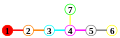one choice of simple roots is given by the rows of the following matrix:### Weyl group

The Weyl group of E7 is of order 2903040: it is the direct product of the cyclic group of order 2 and the unique simple group of order 1451520 (which can be described as PSp6(2) or PSΩ7(2)).

### Cartan matrix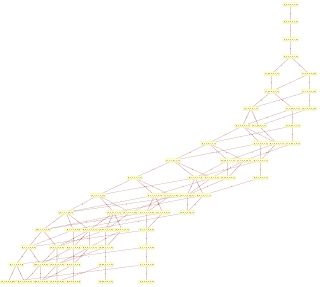Hasse diagram of E7 root poset with edge labels identifying added simple root position## Important subalgebras and representations

E7 has an SU(8) subalgebra, as is evident by noting that in the 8-dimensional description of the root system, the first group of roots are identical to the roots of SU(8) (with the same Cartan subalgebra as in the E7).

In addition to the 133-dimensional adjoint representation, there is a 56-dimensional "vector" representation, to be found in the E8 adjoint representation.

The characters of finite dimensional representations of the real and complex Lie algebras and Lie groups are all given by the Weyl character formula. The dimensions of the smallest irreducible representations are (sequence A121736 in the OEIS):

1, 56, 133, 912, 1463, 1539, 6480, 7371, 8645, 24320, 27664, 40755, 51072, 86184, 150822, 152152, 238602, 253935, 293930, 320112, 362880, 365750, 573440, 617253, 861840, 885248, 915705, 980343, 2273920, 2282280, 2785552, 3424256, 3635840...

The underlined terms in the sequence above are the dimensions of those irreducible representations possessed by the adjoint form of E7 (equivalently, those whose weights belong to the root lattice of E7), whereas the full sequence gives the dimensions of the irreducible representations of the simply connected form of E7. There exist non-isomorphic irreducible representation of dimensions 1903725824, 16349520330, etc.

The fundamental representations are those with dimensions 133, 8645, 365750, 27664, 1539, 56 and 912 (corresponding to the seven nodes in the Dynkin diagram in the order chosen for the Cartan matrix above, i.e., the nodes are read in the six-node chain first, with the last node being connected to the third).

### E7 Polynomial Invariants

E7 is the automorphism group of the following pair of polynomials in 56 non-commutative variables. We divide the variables into two groups of 28, (p, P) and (q, Q) where p and q are real variables and P and Q are 3×3 octonion hermitian matrices. Then the first invariant is the symplectic invariant of Sp(56, R):The second more complicated invariant is a symmetric quartic polynomial:Whereand the binary circle operator is defined by.

An alternative quartic polynomial invariant constructed by Cartan uses two anti-symmetric 8x8 matrices each with 28 components.## Chevalley groups of type E7

The points over a finite field with q elements of the (split) algebraic group E7 (see above), whether of the adjoint (centerless) or simply connected form (its algebraic universal cover), give a finite Chevalley group. This is closely connected to the group written E7(q), however there is ambiguity in this notation, which can stand for several things:

• the finite group consisting of the points over Fq of the simply connected form of E7 (for clarity, this can be written E7,sc(q) and is known as the “universal” Chevalley group of type E7 over Fq),
• (rarely) the finite group consisting of the points over Fq of the adjoint form of E7 (for clarity, this can be written E7,ad(q), and is known as the “adjoint” Chevalley group of type E7 over Fq), or
• the finite group which is the image of the natural map from the former to the latter: this is what will be denoted by E7(q) in the following, as is most common in texts dealing with finite groups.

From the finite group perspective, the relation between these three groups, which is quite analogous to that between SL(n, q), PGL(n, q) and PSL(n, q), can be summarized as follows: E7(q) is simple for any q, E7,sc(q) is its Schur cover, and the E7,ad(q) lies in its automorphism group; furthermore, when q is a power of 2, all three coincide, and otherwise (when q is odd), the Schur multiplier of E7(q) is 2 and E7(q) is of index 2 in E7,ad(q), which explains why E7,sc(q) and E7,ad(q) are often written as 2·E7(q) and E7(q)·2. From the algebraic group perspective, it is less common for E7(q) to refer to the finite simple group, because the latter is not in a natural way the set of points of an algebraic group over Fq unlike E7,sc(q) and E7,ad(q).

As mentioned above, E7(q) is simple for any q, and it constitutes one of the infinite families addressed by the classification of finite simple groups. Its number of elements is given by the formula (sequence A008870 in the OEIS):The order of E7,sc(q) or E7,ad(q) (both are equal) can be obtained by removing the dividing factor gcd(2, q−1) (sequence A008869 in the OEIS). The Schur multiplier of E7(q) is gcd(2, q−1), and its outer automorphism group is the product of the diagonal automorphism group Z/gcd(2, q−1)Z (given by the action of E7,ad(q)) and the group of field automorphisms (i.e., cyclic of order f if q = pf where p is prime).

## Importance in physics

N = 8 supergravity in four dimensions, which is a dimensional reduction from 11 dimensional supergravity, admit an E7 bosonic global symmetry and an SU(8) bosonic local symmetry. The fermions are in representations of SU(8), the gauge fields are in a representation of E7, and the scalars are in a representation of both (Gravitons are singlets with respect to both). Physical states are in representations of the coset E7 / SU(8).

In string theory, E7 appears as a part of the gauge group of one the (unstable and non-supersymmetric) versions of the heterotic string. It can also appear in the unbroken gauge group E8 × E7 in six-dimensional compactifications of heterotic string theory, for instance on the four-dimensional surface K3.